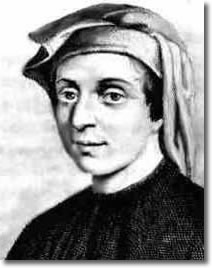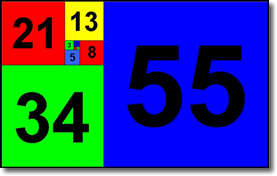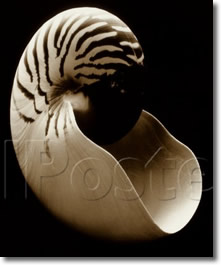# Fibonacci & the Golden Ratio

» While exploring the world of computer programming, I kept running into references to a mathematical concept known as » Fibonacci numbers .. sometimes referred to as the "Fibonacci series" or a "Fibonacci sequence" (of numbers).Leonardo Fibonacci (pronounced » fee-boh-'nah-chee) was an Italian mathematician, born in Pisa (.. that's right, home of the famous leaning tower, whose construction began several years after Leonardo's birth).

He was a true pisan, who lived from 1170 to 1250.

Leonardo's father was Guglielmo Bonacci. The name Fibonacci means 'Son of Bonacci' .. a shortening of the Latin "filius Bonacci" .. similar to the way the name Anderson means 'Son of Ander'.

[ I was surprised to learn that Leonardo never actually used the name Fibonacci. ]

Some references cite him as the "greatest European mathematician of the Middle Ages." His greatest contribution was the introduction to Europe of the Hindu-Arabic decimal number system that we use today, based on ten digits with a positional decimal point and a symbol for zero.

At the time, Europe was still using the clunky Roman Numeral system, which made calculation difficult.

The Fibonacci numbers (or Fibonacci series) were (was) originally derived from a problem to calculate the population growth of rabbits under ideal conditions.See here:

A certain man put a pair of rabbits in a place surrounded on all sides by a wall. How many pairs of rabbits can be produced from that pair in a year if it is supposed that every month each pair begets a new pair which from the second month on becomes productive?

Starting with zero and one, you calculate the series by adding the previous two numbers to get the next. Specifically » 0, 1, 1, 2, 3, 5, 8, 13, 21, 34, 55, 89, 144, 233, 377, 610, 987, 1597, and so forth. In other words, the next number is always the sum of the previous two. Simple, no?

### The Golden Ratio

Perhaps more interesting .. is how we derive a good approximation of the golden ratio by dividing one Fibonacci number by the next (.. either way, up or down).

The golden ratio is defined as roughly 1.618-to-1, or 0.618-to-1 .. which is close to a ratio of one-third to two-thirds (33-to-66), and is usually associated with the Greek letter phi φ.

[ Actually, 60-40 is a closer approximation than one-third, two-third. While 62-to-38 is very close. The actual golden-ratio number is a decimal that goes on forever, so everything is an approximation.

I've divved up the home page according to the golden ratio, with the blue-green sidebars taking roughly 38% of total page width .. at least, when you use the default text size .. seeing the current layout is a liquid-elastic hybrid. ]

••• today's entry continues here below •••The golden ratio often shows up in nature. It is defined as »

.. two quantities are in the golden ratio if the ratio of the SUM of the quantities to the LARGER quantity is equal to the ratio of the LARGER quantity to the SMALLER one.

Might need to ponder that a minute or two (.. many mathematicians & artists have pondered it for hours), but it is talking about two different ratios being equal, the same.

Let's get practical a minute and say, for example, that you're a man who weighs 180 pounds. That means a woman who weighs 111 pounds (180 x 0.618) would fall into your golden ratio.

The golden ratio isnt normally used for things like this; I'm merely trying to give you a feel for the size of the ratio.

I thought the Fibonacci series would be a complicated mathematical concept. The most surprising thing has been how simple it actually is.

Note that Fibonacci introduced the sequence to Europe in 1202, when he published his book titled Book of Calculation. But Indian mathematicians had described the sequence some 6 or 7 centuries earlier. (Centuries, yeah.)

[ Our word algebra comes from the Arabic word » al-jabr, which is taken from the title of a book written by the Persian mathematician al-Khwārizmī (.. from which we derive our word algorithm, which is simply a list of instructions for solving a problem). al-Khwarizmi spent time in India, learning mathematics from the Hindu Indians there. ]

I've always been good at math. Algebra and even Calculus. I enjoy math .. cuz the rules dont change. I've never heard of Fibonacci .. prior to my exploration of programming languages. Cool name, tho.

"How exactly are Fibonacci numbers used in programming?" you ask. I havent gotten to that part yet. =) ■

For more along these lines, here's a Google search preconfigured for the query » fibonacci and another for » fibonacci programming